# Texas Go Math Grade 3 Lesson 14.4 Answer Key Relate Multiplication and Division

Refer to our Texas Go Math Grade 3 Answer Key Pdf to score good marks in the exams. Test yourself by practicing the problems from Texas Go Math Grade 3 Lesson 14.4 Answer Key Relate Multiplication and Division.

## Texas Go Math Grade 3 Lesson 14.4 Answer Key Relate Multiplication and Division

Unlock the Problem

Pamela ordered a set of 6 books written by her favorite author. The set of books cost $48. Since she had already read them, Pamela sold 2 of the books to her friend Jody. If each book was the same price, how much did Jody pay? What operations will you use to solve the problem? Multiplication and division Circle the numbers you need to use. Answer: Example Use strip diagrams. First, complete the strip diagram to show$48 divided into 6 equal groups.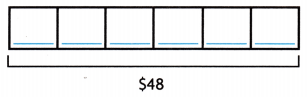Complete the equation.
48 ÷ 6 = _________
So, each book cost $_______. Answer:Complete the equation. 48 ÷ 6 = 8 So, each book cost$ 8.

Then, complete another strip diagram to show the cost of 2 books.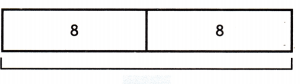Complete the equation.
2 × 8 = _________
So, Jody paid $_________ for the 2 books. What multiplication sentence can you write to solve the division problem? Answer: 2 × 8 =16 So, Jody paid$16 for the 2 books.

Math Talk
Mathematical Processes

What information is given in each strip diagram?

Example Use arrays.

Blair baked 4 batches of 9 cupcakes. He packed the cupcakes in 6 equal boxes. How many cupcakes did he put in each box?

Show an array with 9 counters in 4 equal rows by completing the drawing.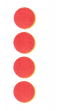There are _________ counters.
Complete the equation. 4 × 9 = _________
So, Blair baked _________ cupcakes.
Explanation:There are 9 counters.
Complete the equation. 4 × 9 = 36
So, Blair baked 36 cupcakes.

Now, complete the array to show the number of cupcakes in each box.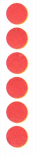There are _________ counters in each row.
Complete the equation. 36 = 6 = _________
So, there are _________ cupcakes in each box.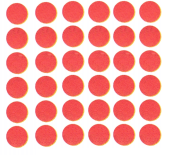There are 6 counters in each row.
Complete the equation. 36 = 6 x 6
So, there are 6 cupcakes in each box.

Share and Show

The third grade students sat in 6 seats at 5 tables in the lunchroom. Show how the same number of students can sit in equal groups at 3 tables.

Question 1.
Complete the strip diagram to show the total number of students.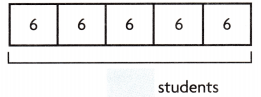Complete the equation.
5 × 6 = _________
So, there are _________ third grade students.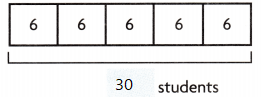Complete the equation.
5 × 6 = 30
So, there are 30 third grade students.

Math Talk
Mathematical Processes

Why are two steps needed to solve this problem?
To find the equation.

Question 2.
Complete the strip diagram to show the number of students at 3 tables.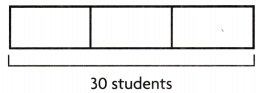Complete the equation.
30 ÷ 3 = _________
So, there are _________ students at each table.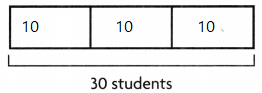Completed the equation.
30 ÷ 3 = 10
So, there are 10 students at each table.

Problem Solving

Use the table for 3-5. You may use arrays, strip ‘diagrams, and equations to represent and solve.Question 3.
Mr. Patterson paid $24 for some students to get into the fair. How many students did Mr. Patterson pay for? Answer: 8 Students Explanation: Mr. Patterson paid$24 for some students to get into the fair.
For 8 students  Mr. Patterson payed

Question 4.
H.O.T. Multi-Step Garrett is 8 years old. He and his family are going to the county fair. What is the price of admission for Garrett, his 2 parents, and baby sister?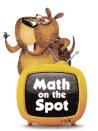Answer: $15 Explanation: Garrett is 8 years old. He and his family are going to the county fair. What is the price of admission for Garrett, his 2 parents, For student 3$
For adult 6$3 + 12 = 15$

Question 5.
H.O.T. Multi-Step Jose and Izzy are 8 years old. They go to the fair on a day when all rides are $2 and admission is$1 off. How much will they pay altogether to attend the fair and go on 5 rides each?
Answer: $15 Explanation: Jose and Izzy are 8 years old. 2 x 3$ = $6 They go to the fair on a day when all rides are$2 and admission is $1 off. 6 – 1 = 5$
How much will they pay altogether to attend the fair and go on 5 rides each.
5 x 2 = $10 10 + 5 = 15$

Question 6.
There are 20 seats on the Wildcat ride. The number of seats in each car is the same. If there are 5 cars on the ride, how many seats are there in each car? Complete the strip diagram to show the problem. Then answer the question.Explanation:
4 seats are there in each car
Completed the strip

Question 7.
Write Math Pose a Problem How many days are there in 2 weeks? Write and solve a related word problem to represent the inverse operation.
Explanation:
A week has 7 days
2 weeks x 7 = 14
A week has 14 days
14 ÷ 2 = 7
The two operations are inverse operations2 weeks of _______ = 14
2 × _______ = 14
14 ÷ 2 = _________
2 row of 7 = 14
2 × 7 = 14
14 ÷ 2 = 7

Fill in the bubble for the correct answer choice. You may use arrays, strip diagrams, and equations to represent and solve.

Question 8.
Multi-Step Paul is in charge of the egg toss at the World Egg Day fair. There are 48 people participating in teams of 8 people. Each team needs 1 egg. Paul is buying eggs in cartons that each have 6 eggs. How many cartons does Paul need?
(A) 1
(B) 2
(C) 8
(D) 6
Explanation:
Paul is in charge of the egg toss at the World Egg Day fair.
There are 48 people participating in teams of 8 people.
Each team needs 1 egg.
Paul is buying eggs in cartons that each have 6 eggs.
48 ÷  6 = 8
Paul need 8 cartons.

Question 9.
Apply Kat is reading a book with 36 pages. She wants to finish the book in 4 days. How many pages does she need to read each day?
(A) 8
(B) 6
(C) 4
(D) 9
Explanation:
Kat is reading a book with 36 pages.
She wants to finish the book in 4 days.
9 pages  she need to read each day.
36 ÷ 4 = 9

Question 10.
Multi-Step Roshan has 18 crackers. He shares the crackers equally with his brother. Then Roshan eats three crackers. How many crackers does Roshan have left?
(A) 21
(B) 15
(C) 6
(D) 9
Explanation:
Roshan has 18 crackers.
He shares the crackers equally with his brother.
18 ÷  2 = 9
Then Roshan eats three crackers.
9 – 3 = 6
Roshan have left 6 crackers

Texas Test Prep

Question 11.
Use Tools There are 35 prizes in 5 equal rows. How many prizes are in each row?
(A) 9
(B) 7
(C) 8
(D) 6
Explanation:
There are 35 prizes in 5 equal rows.
7 x 5 = 35.
so, Each row has 7 prizes

### Texas Go Math Grade 3 Lesson 14.4 Homework and Practice Answer Key

Complete the equation.

Question 1.2 row of _______ = 14
2 × _______ = 14
14 ÷ 2 = _________
Explanation:
Inverse operation is used to find
2 row of 7 = 14
2 × 7 = 14
14 ÷ 2 = 7

Question 2.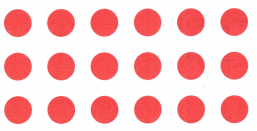3 row of _______ = 18
3 × _______ = 18
18 ÷ 3 = _________
Explanation:
Inverse operation is used to find

Question 3.
6 × ______ = 24
24 ÷ 6 = ________
Explanation:
Inverse operation is used to find
6 × 4 = 24
24 ÷ 6 = 4

Question 4.
8 × ______ = 56
56 ÷ ________ = 8
Explanation:
Inverse operation is used to find
8 × 7 = 56
56 ÷ 7 = 8

Question 5.
8 × ______ = 64
64 ÷ 8 = ________
Explanation:
Inverse operation is used to find
8 × 8 = 64
64 ÷ 8 = 8

Question 6.
5 × ______ = 45
45 ÷ 5 = ________
Explanation:
Inverse operation is used to find
5 × 9 = 45
45 ÷ 5 = 9

Problem Solving

Use the table for 7-10. You may use arrays, strip diagrams, and equations to represent and solve.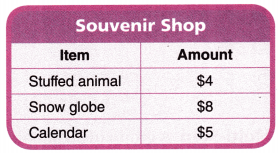Question 7.
Harry pays $16 for stuffed animals. How many stuffed animals does Harry buy? Answer: 4 Animals Explanation: According to the table 1 stuffed animal =$4
16 ÷ 4 = 4

Question 8.
Mrs. Kahn buys 3 snow globes for her children. How much money does Mrs. Kahn spend on the snow globes?
Explanation:
1 snow globe cost is $8 Mrs. Kahn buys 3 snow globes for her children. 8 x 3 =$24
$24 Mrs. Kahn spend on the snow globes Question 9. On Tuesdays, stuffed animals and calendars are each$1 off. How much will Marcus pay if he waits until Tuesday to buy 3 stuffed animals and 2 calendars?
According to the table 1 stuffed animal = $4 one off is 4 – 1 =$3
According to the table 1 calendar= $5 one off is 5 – 1 =$4
Marcus pay if he waits until Tuesday to buy 3 stuffed animals and 2 calendars
3 x 3 + 4 x 2
9 + 8 = 17$Lesson Check Fill in the bubble completely to show your answer. You may use arrays, strip diagrams, and equations to represent and solve. Question 10. Ned earns$15 delivering newspapers. He earns $5 each day. How many days does Ned deliver newspapers? (A) 20 days (B) 5 days (C) 3 days (D) 10 days Answer: C Explanation: Ned earns$15 delivering newspapers.
He earns $5 each day. 3 days Ned deliver newspapers 15 ÷ 5= 3 Question 11. Janella buys some comic books. She spends$12. Each comic book costs $2. How many comic books does Janella buy? (A) 14 (B) 10 (C) 5 (D) 6 Answer: D Explanation: Janella buys some comic books. She spends$12.
Each comic book costs \$2.
12 ÷  2 = 6
6 comic books does Janella buy

Question 12.
There are 28 children going on a scavenger hunt. They need to make teams of 4. How many children are on each team for the scavenger hunt?
(A) 7
(B) 8
(C) 6
(D) 5
Explanation:
There are 28 children going on a scavenger hunt.
They need to make teams of 4.
8 children are on each team for the scavenger hunt
28 ÷  4 = 8

Question 13.
Mr. Brown wants to build a fence that measures 36 feet long. Each section of fencing is 6 feet long. How many sections of fence does Mr. Brown need?
(A) 30
(B) 42
(C) 9
(D) 6
Explanation:
Mr. Brown wants to build a fence that measures 36 feet long.
Each section of fencing is 6 feet long.
36 ÷  6 = 6
6 sections of fence does Mr. Brown need

Question 14.
Multi-Step A group of 30 people are going to the zoo. There are 4 cars. If each car seats 5 people, how many more cars will they need for the trip?
(A) 10
(B) 2
(C) 20
(D) 5
Explanation:
A group of 30 people are going to the zoo.
There are 4 cars. If each car seats 5 people,
4 x 5 = 20
30 – 20 = 10
2 x 5 = 10
2 more cars will they need for the trip

Question 15.
Multi-Step Paula has 21 shells she collected at the beach. She shares them equally with herself and 2 friends. Then she gives 3 shells to her mother. How many shells does Paula have left?
(A) 7
(B) 4
(C) 3
(D) 5